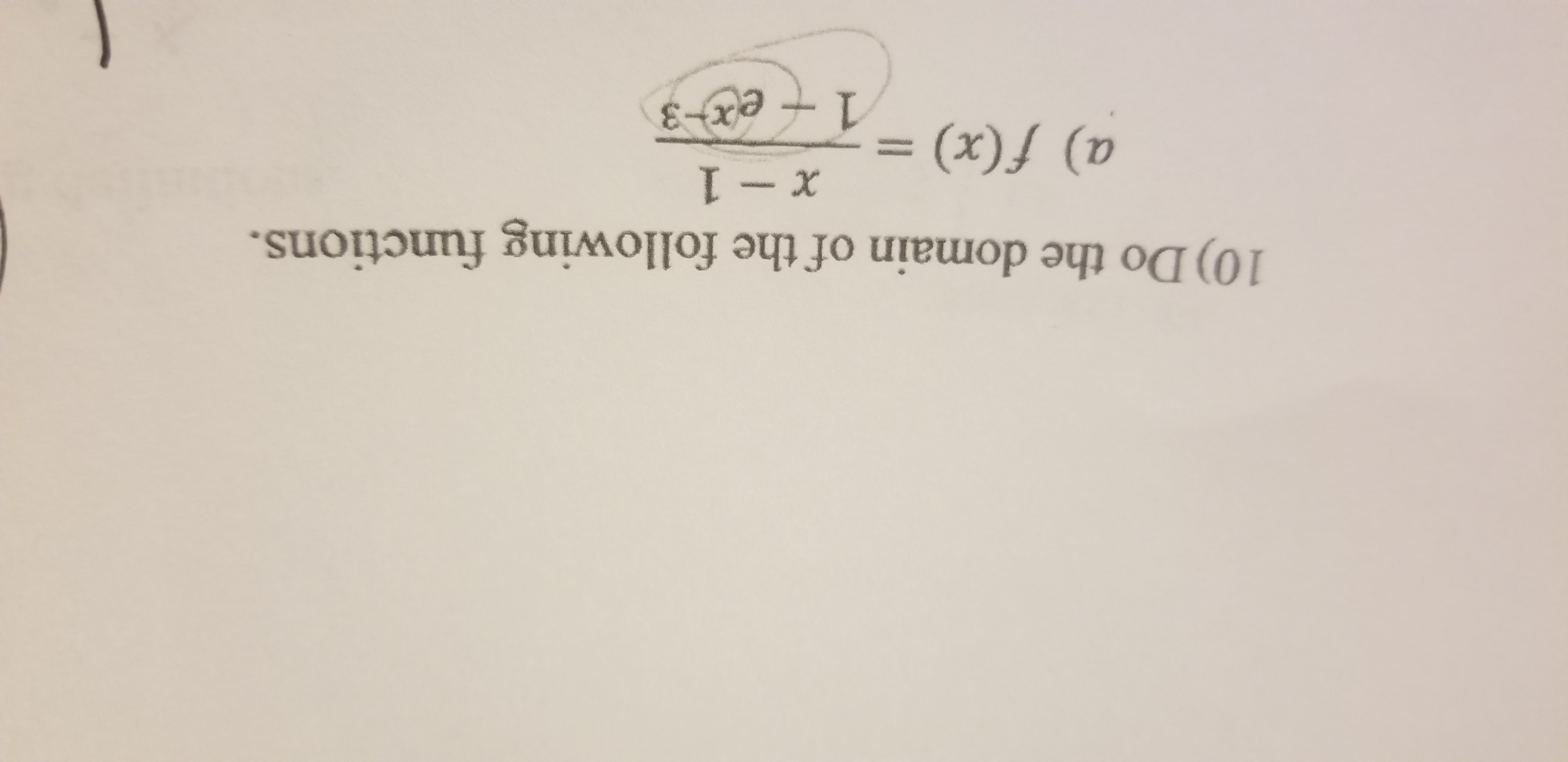# 10) Do the domain of the following functions.a) f(x) =1+e&

Question
1 views

Do the domain of the following function:help_outlineImage Transcriptionclose10) Do the domain of the following functions. a) f(x) = 1+e& fullscreen
check_circle

Step 1

Consider the provided function.

Step 2

The domain of a function is the set of input for which the function is real and defined.

Step 3

To find the domain of a function, set the denominator equal to zero and exclude the value of x that you find afte...

### Want to see the full answer?

See Solution

#### Want to see this answer and more?

Solutions are written by subject experts who are available 24/7. Questions are typically answered within 1 hour.*

See Solution
*Response times may vary by subject and question.
Tagged in

### Calculus